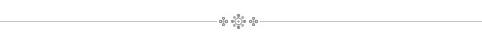## Where We Find It : Mathematics### The Math You Used to Know (Or Maybe Never Did)

Categories: Mathematics

S.O.S. Mathematics is a great site for short, informative introductions to all sorts of basic mathematics.

S.O.S. MATHematics is your free resource for math review material from Algebra to Differential Equations!

The perfect study site for high school, college students and adult learners. Get help to do your homework, refresh your memory, prepare for a test, ....

Browse our more than 2,500 Math pages filled with short and easy-to-understand explanations. Click on one of the following subject areas: Algebra, Trigonometry, Calculus, Differential Equations, Complex Variables, Matrix Algebra, or Mathematical Tables.

You can find topics ranging from simplifying fractions to the cubic formula, from the quadratic equation to Fourier series, from the sine function to systems of differential equations - this is the one stop site for your math needs.

Be the first to Comment | Permalink